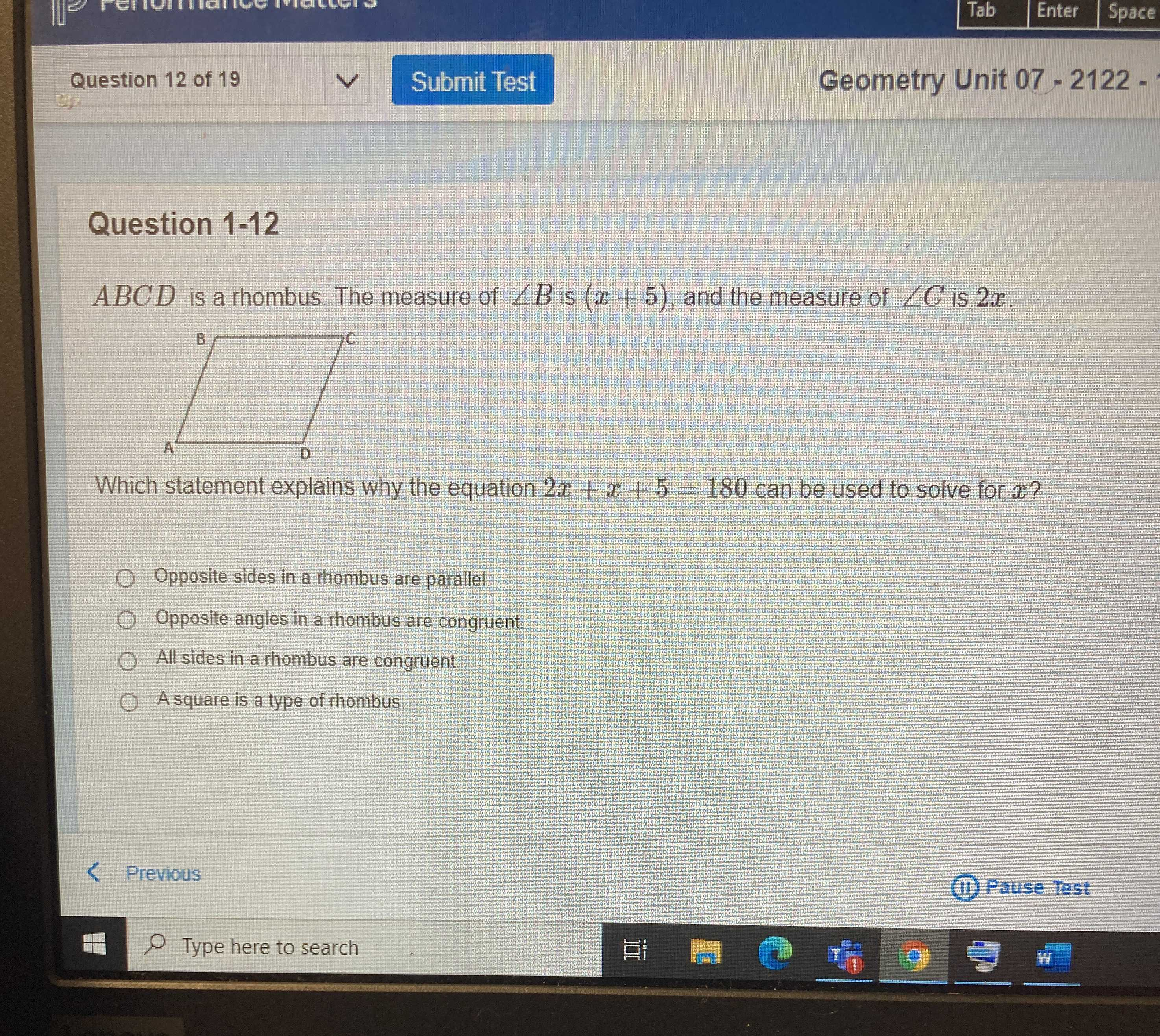### ¿Todavía tienes preguntas de matemáticas?

Pregunte a nuestros tutores expertos
Algebra
Pregunta$$A B C D$$ is a rhombus. The measure of $$\angle B$$ is $$( x + 5 )$$ , and the measure of $$\angle C$$ is $$2 x$$ .

Which statement explains why the equation $$2 x + x + 5 = 180$$ can be used to solve for $$x ?$$ Opposite sides in a rhombus are parallel.

Opposite angles in a rhombus are congruent.

All sides in a rhombus are congruent.

Asquare is a type of rhombus.